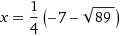# Can You Solve 2x^2+7x=5?thanked the writer.
Let's solve the quadratic equation:
2x^2+7x = 5
First we need to put the equation in the form:
ax^2 + bx + c = 0
For this, we set the equation to equal 0, and arrange the terms from the highest exponent to the lowest:
2x^2+7x-5 = 0
We have:
a=2 b=7 c=-5
We can now apply the quadratic formula:
x = ( -b ± √(b²-4ac) ) / 2a
We find two solutions:
x=0.60849528301415 or x=-4.1084952830142

OR

First we need to put the equation in the form:
ax^2 + bx + c = 0
For this, we set the equation to equal 0, and arrange the terms from the highest exponent to the lowest:
2x^2+7x-5 = 0
We have:
a=2 b=7 c=-5
We can now apply the quadratic formula:
x = ( -b ± v(b²-4ac) ) / 2a
We find two solutions:
x=0.60849528301415 or x=-4.1084952830142
thanked the writer.
Subtract 5 from each side, then...
Factor it out
(2x+3)(x-2)
seperate and solve for two possible answers
2x+3=0, where x=-3/2
x-2=0, where x=2
thanked the writer.
rajeev dandu commented
This is so simple and correct.
2x2 + 7x=5

Or, 2x2 + 7x-5=0

here,
a=2 b=7 c=-5

applying  the quadratic formula-

x = ( -b ± √(b²-4ac) ) / 2a

x=0.60849528301415   or   x=-4.1084952830142
thanked the writer.